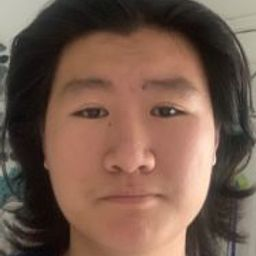🤔 Find out what you don't know with free Quizzes 🤔Start Quiz Now!# Evaluate $\int_{C} \frac{1}{x^{2}+y^{2}+1} d s,$ where $C$ is given in the accompanying figure. (FIGURE CAN'T COPY)

Integrals

Vectors

Vector Functions

### Discussion

You must be signed in to discuss.
##### Top Calculus 3 Educators##### Catherine R.

Missouri State University##### Michael J.

Idaho State University

Lectures

Join Bootcamp

### Video Transcript

Okay, folks. So in this video, we're going to take a look at problem number 26. Um, in this problem, we have been given a graph. Ah, graphical curve C, which is basically just a square. Um, we're integrating a function along the square. So what we're going to Dio is we're going to take the strategy where we kind of no break the square up into four separate pieces. Um, so we're first going to be integrating along this curve, and then we're going up, and then we're going this way, and then we're going back to where we started from. So that's our strategy. So let's write it out. We have Ah, I'm to call this thing. So you want And this things you to win this thing. For some reason, my pen is not working. This is the three indecency for Okay, So see you one into Gro plus C two into girl plus See three integral plus C four, integral. Okay, so now let's write them all out. We have ah for C one. If you notice something is not changing everywhere along the currency One. What is that thing? That thing is why? Why it's not changing. Why has the constant fixed value of zero So for the function, I'm just going to write one over X squared. Plus what DX I'm supposed to write the s here, but the S is the same thing as DX because I'm not varying. Why? And X, of course, is going from zero all the way to one and then for the second Integral, um, X is not changing. So I can write. DS is d Y and X as a constant value of one. So I'm just gonna write one over y squared, plus two more light by the Why why goes from 0 to 1. So that's the second into grow. As for the third, integral, we're going to be doing, uh, one over, as you can see why it's not changing again and why this time has a constant value of one. So we have X squared plus two GX and ex is going from one back to zero close. So for the fourth into grow, we have ah, you know, we have a constant value for X, which is zero. So I'm going to write one over y squared, plus one for the function if multiplied by the yes, which is the same thing as you know you. Why? Because exits not changing. So we have d why here and why is going from one 20? Okay, this thing right here, I want to remind you You remember this thing right here is the Arc Tangent. I don't want to write that many letters. I was gonna write this as tan universe effects between zero and one. And this thing right here is tan in various before I from 120 Okay. And this and this is a little bit trickier, so we're gonna have to do them separately. Um, this thing right here if I if I drag it here, I have 01 1/2 one over one. Plus, why? I swear it over to Okay. I just did a little bit of outbreak manipulation here, which is nothing profound. I pulled out a constant factor if want half, um, which you can always do because you can always pull out of constant factor. Um, I'm gonna pull that out of the integral 0 to 11 over. Why squared over to, um Now I'm gonna do something very very interesting. Not that interesting, but, you know, I'm gonna write. Why squared over two as defined to be you. Basically, I'm defining the U substitution here. So the you is Ah, 1/2 times to why do you Why we just why do you Why? Okay, so ah.University of California, Berkeley

#### Topics

Integrals

Vectors

Vector Functions

##### Top Calculus 3 Educators##### Catherine R.

Missouri State University##### Michael J.

Idaho State University

Lectures

Join Bootcamp